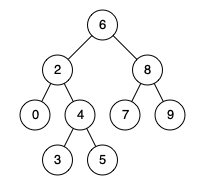# 235. Lowest Common Ancestor of a Binary Search Tree (Easy)

https://leetcode.com/problems/lowest-common-ancestor-of-a-binary-search-tree/

Given a binary search tree (BST), find the lowest common ancestor (LCA) of two given nodes in the BST.

According to the definition of LCA on Wikipedia: “The lowest common ancestor is defined between two nodes p and q as the lowest node in T that has both p and q as descendants (where we allow a node to be a descendant of itself).”

Given binary search tree:  root = [6,2,8,0,4,7,9,null,null,3,5]Example 1:

```Input: root = [6,2,8,0,4,7,9,null,null,3,5], p = 2, q = 8
Output: 6
Explanation: The LCA of nodes `2` and `8` is `6`.
```

Example 2:

```Input: root = [6,2,8,0,4,7,9,null,null,3,5], p = 2, q = 4
Output: 2
Explanation: The LCA of nodes `2` and `4` is `2`, since a node can be a descendant of itself according to the LCA definition.
```

Note:

• All of the nodes' values will be unique.
• p and q are different and both values will exist in the BST.

## Solutions

### 1.

``````class Solution {

public TreeNode lowestCommonAncestor(TreeNode root, TreeNode p, TreeNode q) {
if (root == null) {
return null;
}

List<TreeNode> pTrack = new ArrayList<>();
List<TreeNode> qTrack = new ArrayList<>();

// Get the track to p and q nodes
searchNode(root, p, pTrack);
searchNode(root, q, qTrack);

int lenp = pTrack.size();
int lenq = qTrack.size();

int len = Math.min(lenp, lenq);

// Find the first different node before which is the lowest common ancestor.
for (int i = 0; i < len; i++) {
if (pTrack.get(i).val != qTrack.get(i).val) {
return pTrack.get(i - 1);
}
}

// Certain one is the prefix of another.
return pTrack.get(len - 1);
}

private void searchNode(TreeNode root, TreeNode x, List<TreeNode> track) {

if (root == null) {
return;
}

if (root.val == x.val) {
return;
}

if (root.val > x.val) {
searchNode(root.left, x, track);
} else {
searchNode(root.right, x, track);
}
}
}
``````

### 2.

``````class Solution {

public TreeNode lowestCommonAncestor(TreeNode root, TreeNode p, TreeNode q) {
// Value of current node or parent node.
int parentVal = root.val;

// Value of p
int pVal = p.val;

// Value of q;
int qVal = q.val;

// If both p and q are greater than parent
if (pVal > parentVal && qVal > parentVal) {
return lowestCommonAncestor(root.right, p, q);
}

// If both p and q are lesser than parent
if (pVal < parentVal && qVal < parentVal) {
return lowestCommonAncestor(root.left, p, q);
}

// We have found the split point, i.e. the LCA node.
return root;
}
}
``````